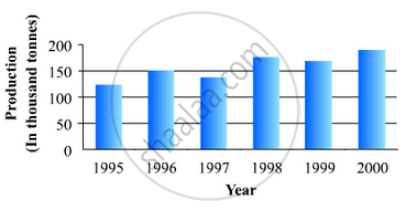# The following data gives the production of foodgrains (in thousand tonnes) for some years: Represent the above with the help of a bar graph. - Mathematics

The following data gives the production of foodgrains (in thousand tonnes) for some years:

 Year 1995 1996 1997 1998 1999 2000 Production(in thousand tonnes 120 150 140 180 170 190

Represent the above with the help of a bar graph.

#### Solution

To represent the given data by a vertical bar graph, we first draw horizontal and vertical axes. Let us consider that the horizontal and vertical axes represent the years and the production of food grains in thousand tonnes respectively. We have to draw 6 bars of different lengths given in the table.

At first we mark 6 points in the horizontal axis at equal distances and erect rectangles of the same width at these points. The heights of the rectangles are proportional to the production of food grains.

The vertical bar graph of the given data is following:Concept: Graphical Representation of Data
Is there an error in this question or solution?

#### APPEARS IN

RD Sharma Mathematics for Class 9
Chapter 23 Graphical Representation of Statistical Data
Exercise 23.2 | Q 10 | Page 26

Share